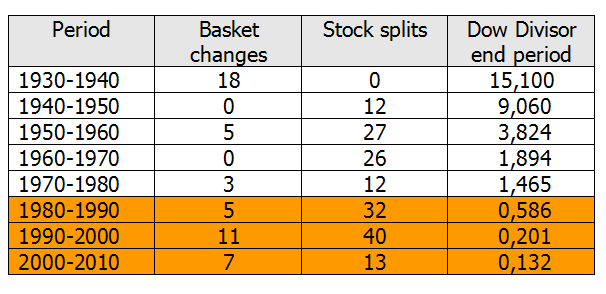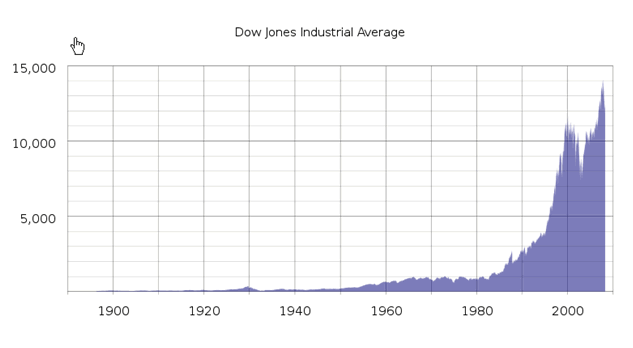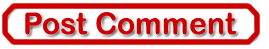Best of the WeekWill Obama Manipulate the Dow Divisor, from Fiction to Reality?

Politics / Market Manipulation Sep 03, 2012 - 07:45 AM GMT

By: SubmissionsWim Grommen writes: Dow Jones Index to 58 points

After intensive consultation with his advisors, President Obama has decided to modify the formula used for calculating the Dow Jones Index. On January 1, 2013 the Dow Divisor will be changed to 30. The result of this change will be that the Dow Jones Index drops from the current 13,124 points to about 58 points. The underlying value of the 30 component stocks of course remains unchanged. With this modification to the formula Obama aims to make the large Dow fluctuations of recent decennia a thing of the past, and hopes that this will bring stability to the stock market. Banks, major investors and pension funds welcome this change in the Dow Jones Index formula.

To understand Obama’s proposal we must go back in Dow Jones history. The Dow was first published in 1896. The Dow was calculated by dividing the sum of the 12 component company stocks by 12:

Dow-index_1896 = (x1 + x2+ ..........+x12) / 12

In 1916 the Dow was enlarged to 20 companies; 4 were removed and 12 added:

Dow-index_1916 = (x1 + x2+ ..........+x20) / 20

The shares of a number of companies were split in 1927, and for those shares a weighting factor was introduced in the calculation. The formula is now as follows

(x1 = American Can is multiplied by 6, x2 = General Electric by 4 etc. )

Dow-index_1927 = (6.x1 + 4.x2+ ..........+x20) / 20

On 1 October 1928 the Dow was further enlarged to 30 stocks. Because everything had to be calculated by hand, the index calculation was simplified. The Dow Divisor was introduced. The index was calculated by dividing the sum of the share values by the Dow Divisor. In order to give the index an uninterrupted graph the Dow Divisor was given the value 16.67.

Dow-index_Oct_1928 = (x1 + x2+ ..........+x30) / Dow Divisor

Dow-index_Oct_1928 = (x1 + x2+ ..........+x30) / 16.67

Since then the Dow Divisor has acquired a new value every time there has been a change in the component stocks, with a consequent change in the formula used to calculate the index. This is because at the moment of change the results of two formulas based on two different share baskets must give the same result. When stocks are split the Dow Divisor is changed for the same reason.

In autumn 1928 and spring 1929 there were 8 stock splits, causing the Dow Divisor to drop to 10.47.

Dow-index_Sep_1929 = (x1 + x2+ ..........+x30) / 10.47

After the stock market crash of 1929, 18 companies were replaced in the Dow and the Dow Divisor got the value 15.1.Table: Changes in the Dow, stock splits and Dow Divisor

The table above makes it clear that the Dow Jones formula has been changed many times and that the Dow Divisor in the period 1980-2010 has actually become a Dow Multiplier, due to the large number of stock splits in that period. Where in the past the sum of the share values was divided by the number of shares, nowadays the sum of the share values multiplied by 7.5. Dividing by 0.132 is after all the same as multiplying by 7.5. (1 / 0,132 = 7,5). This partly explains the behaviour of the Dow graph since 1980.Figure: the Dow Jones since 1896

The proposed change on 1 January 2013 restores the formula to what it originally was: the sum of the share values divided by the number of component shares.

Dow-index_Jan_2013 = (x1 + x2+ ..........+x30) / 30

The aggregate current value of the 30 component stocks is 1736 dollars. By changing the Dow Divisor from 0,132319125 to 30 (a factor 227 larger) the index will, at current values, change from 13124 to 58 points (a factor 227 smaller).

The emotional factor in the current formula,  the nervousness or euphoria of investors resulting from large fluctuations in the Dow, will be greatly reduced by this change (227 times) and so rationality should gain the upper hand in investor’s decisions.

Obama’s proposed change must still be approved by House and Senate. Both Republicans and Democrats have told Obama they support his proposal. Politicians, irrespective of political colour, desire stability in the stock markets. For the investor however it will take some getting used to: the Dow Jones at 58 points.

By Wim Grommen

wgrommen@hotmail.nl.

The Netherlands